# Category: Machine Learning### Deep Learning – Backpropagation Algorithm Basics

Backpropagation Algorithm – An important mathematical tool for making better and high accuracy predictions in machine learning. This algorithm uses supervised learning methods for training Artificial Neural Networks. The whole idea of training multi-layer perceptrons is to compute the derivatives of the error function or gradient descent concerning weights using the backpropagation algorithm. This algorithm is actually based on the linear algebraic operation with a goal of optimising error function by harnessing its intelligence and provisioning updates.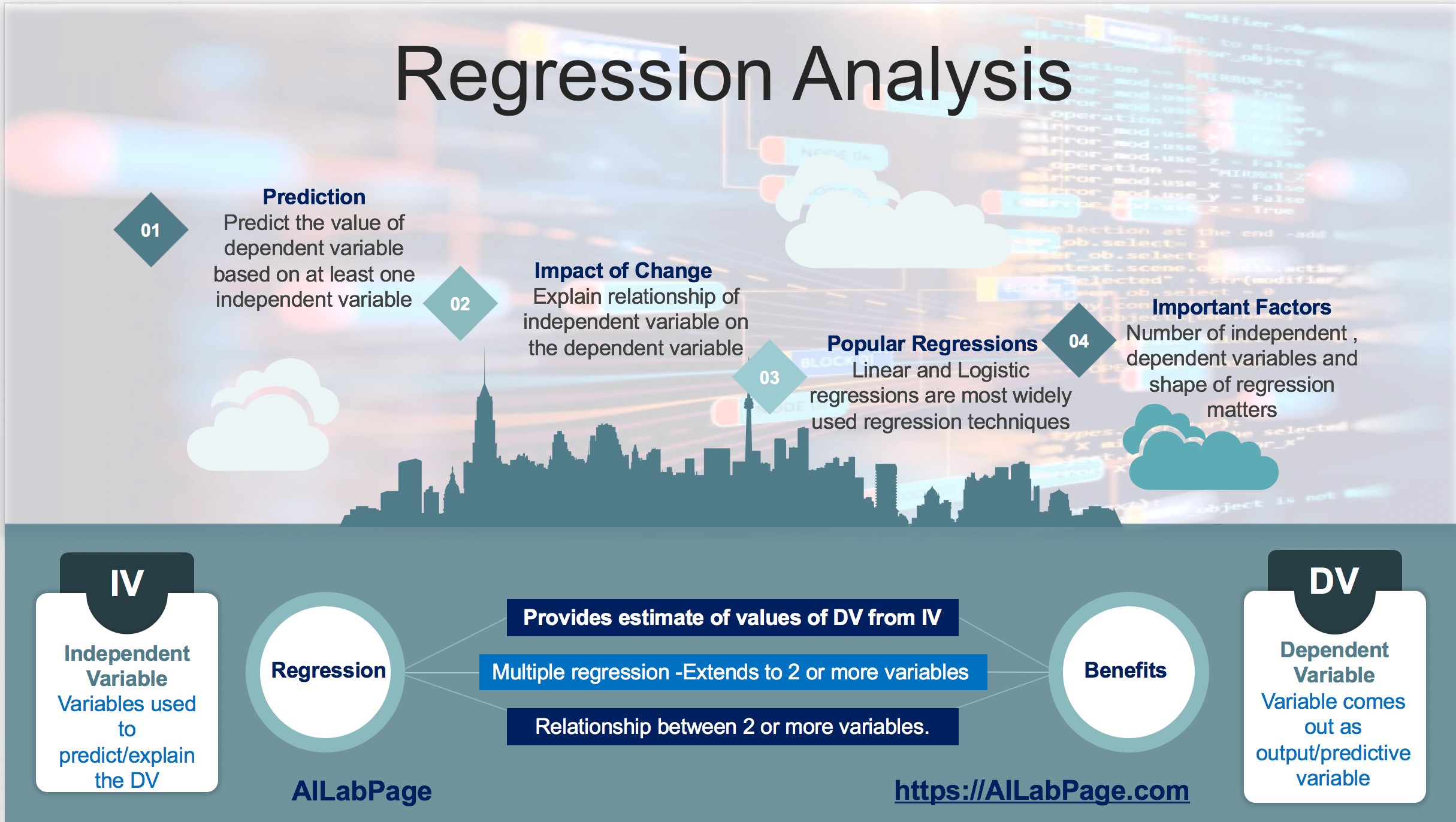### Machine Learning – Introduction to Regression Analysis

In Machine Learning regression analysis is used just to understand how to establish a relationship between independent variables and dependent variables. How independent variables going to effect dependent variables. Whether there is a strong relationship or just a casual relationship between IV and DV. Machine learning is a goal not a technique in this regard while linear regression is. ML can be achieved through many different means and techniques. In short, we can say this### Machine Learning – It all Boils Down to the Training Data

Data generation sources like social media as 1st and winners are doing an excellent job. 2nd to this is payment data which is as big as social media or the Western world. Payments on mobile for e-commerce, online food orders, etc are almost 30 – 50 times more than in the U.S. as in Africa and Asia combined above. Off-course all this data is quality data for making more money as well as to improve the user experience. Data is also used as a yardstick for comparing algorithms.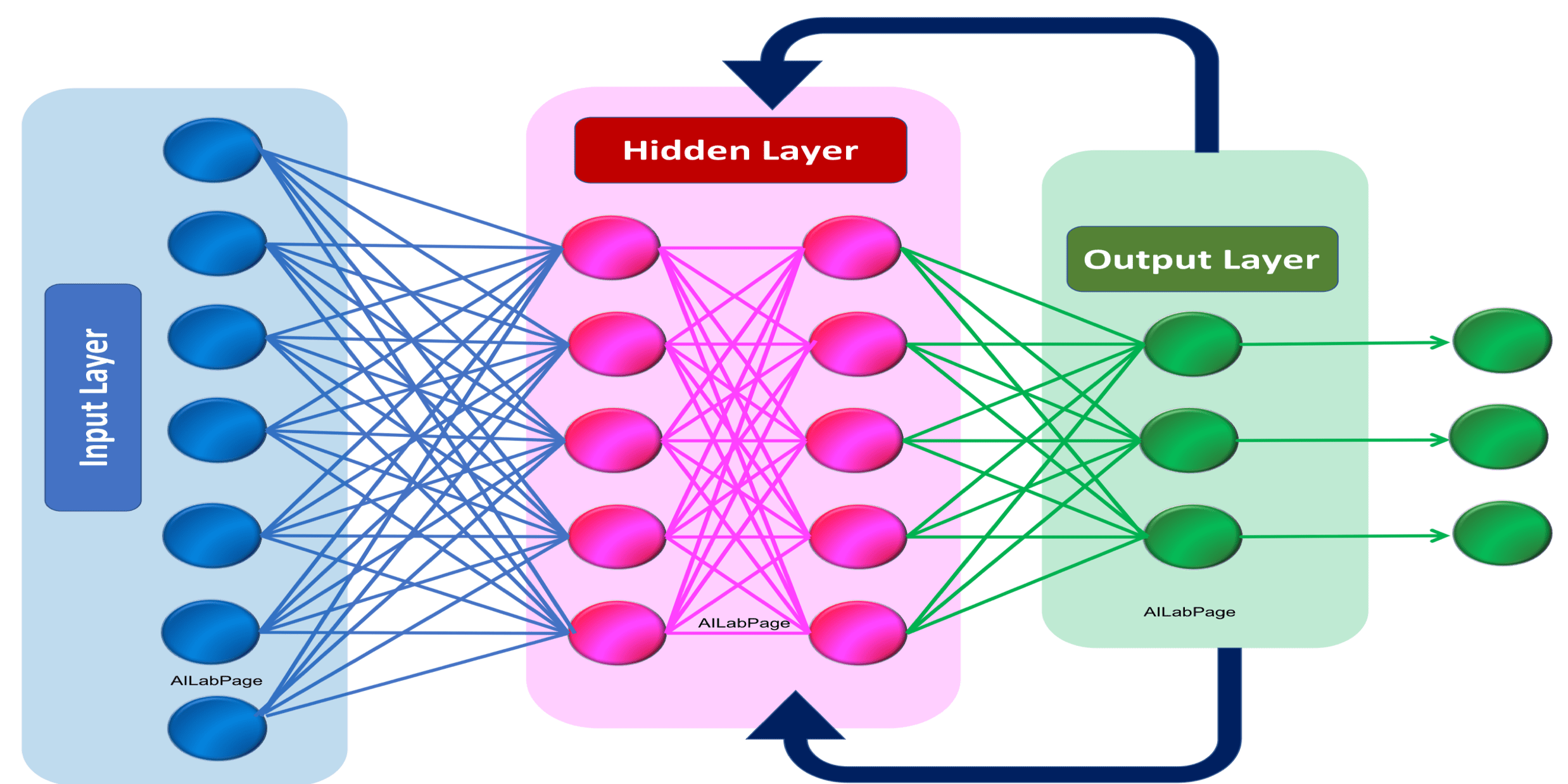### Deep Learning – Introduction to Recurrent Neural Networks

“Artificial neural networks (ANNs) are biologically inspired computing code with number of simple, highly interconnected processing elements for simulating human brain workings to process information model”.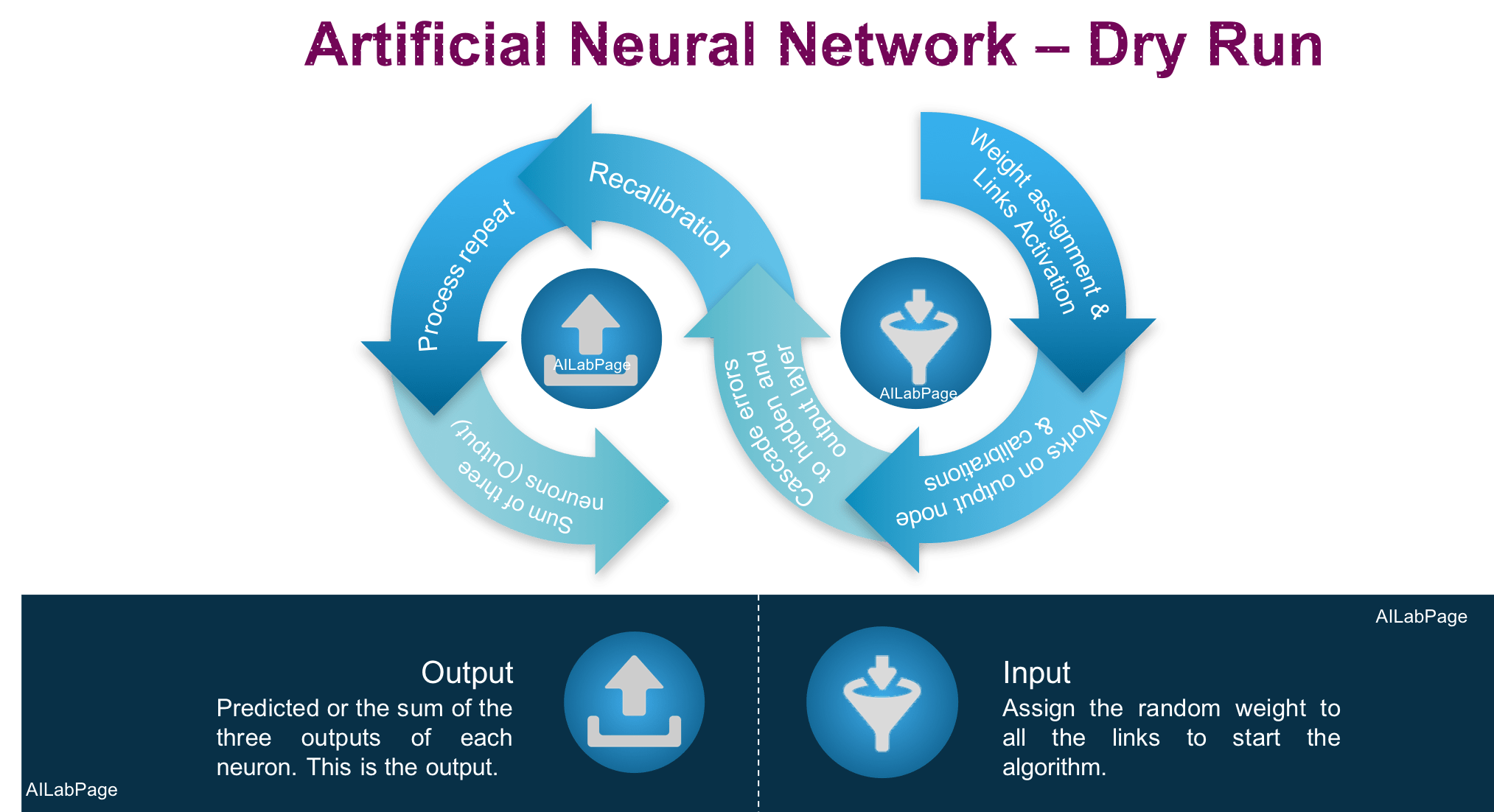### How Neural Network Algorithms Works: An Overview

Artificial neural networks are a type of computing model that takes inspiration from the structure and function of the neural networks found in the human brain. Nevertheless, machine learning has yet to attain authentic biological accuracy, given the present level of implementation and utilization. The process entails receiving multiple inputs and generating a single output.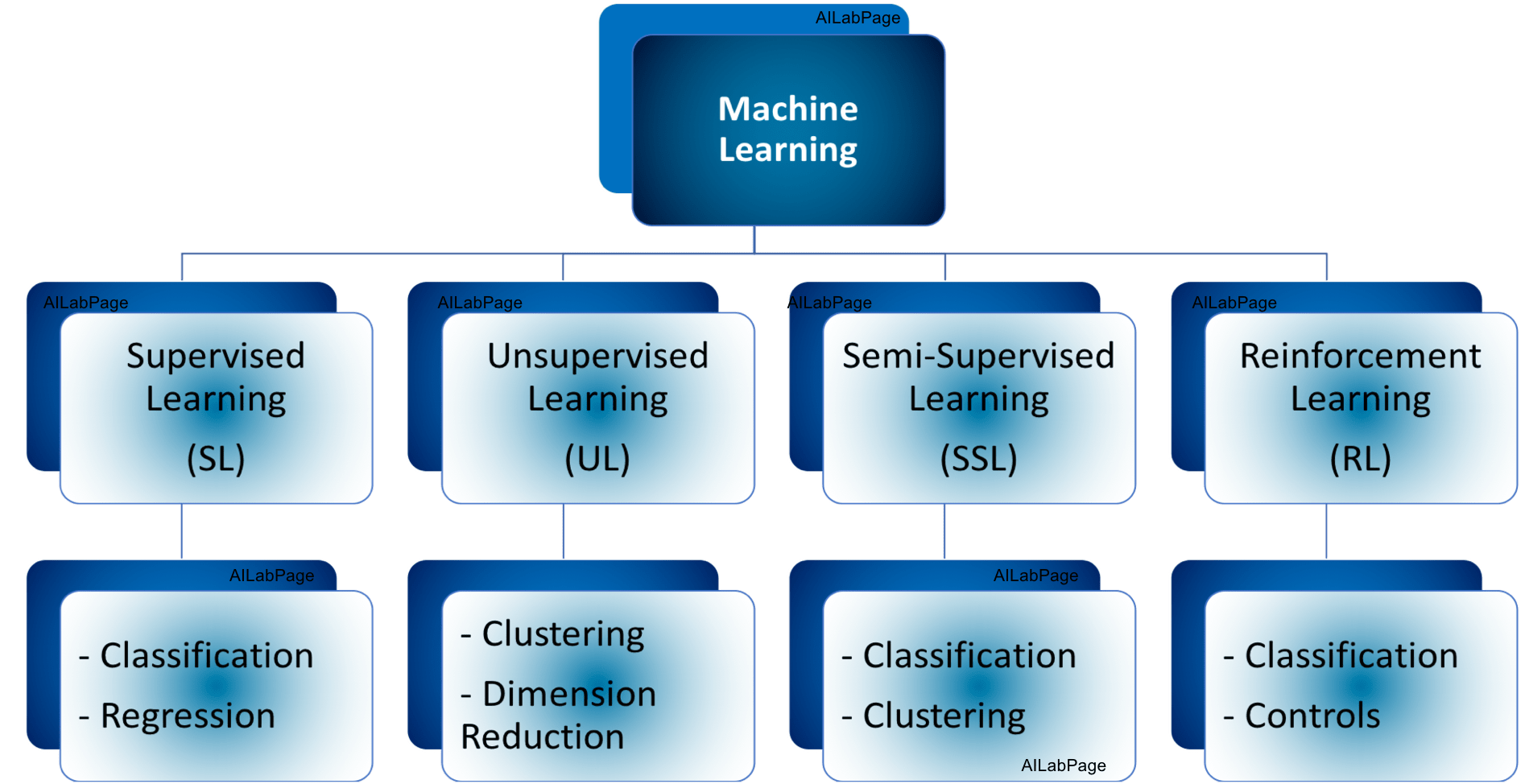### Machine Learning(ML) – Introduction to Basics

Machine learning techniques are accelerating almost daily to bring good value to the businesses of today. It is revolutionizing the way we do our business and what should be done to improve upon it. ML develops its own encompassing strategy from the experience it comes across over the period.### Deep Learning – Introduction to Generative Adversarial Networks (GANs)

GANs consist of two neural networks i.e. Generator that generates a fake image of our currency note example and a disa criminator that classifies it into real or fake. The generator’s role is to map the input to the desired data space (image as in the example above). On the other hand second neural network models i.e. the discriminator classify the output with probability as real or fake compared with real datasets.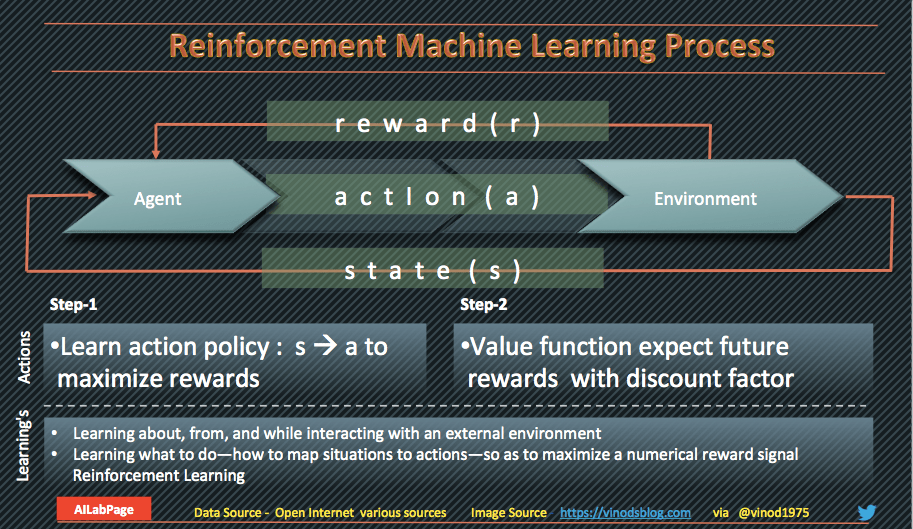### Machine Learning – Introduction to Reinforcement Learning

Reinforcement learning is closely related to dynamic programming approaches to Markov decision processes (MDP). MDP solve a partially observable problem. POMDPs received a lot of attention in the reinforcement learning community. As its a process of discrete-time stochastic control to provide a mathematical framework for decision-making modelling.### Naive Bayes Algorithm – The basics you need to know

The naive Bayes algorithm is a method set of probabilities. For each attribute from each class set, it uses probability to make predictions. This algorithm falls under a supervised machine-learning approach. The data model that comes out of this effort is called as “Predictive Model” with probabilistic problems at the foundation level### Classification and Regression Demystified in Machine Learning

Classification predictive output is a label and for regression its a quantity. Generative algorithms can also be used as classifiers. It just so happens that they can do more than categorising the input data. Can call classification as sorting and regression as connecting technique as well.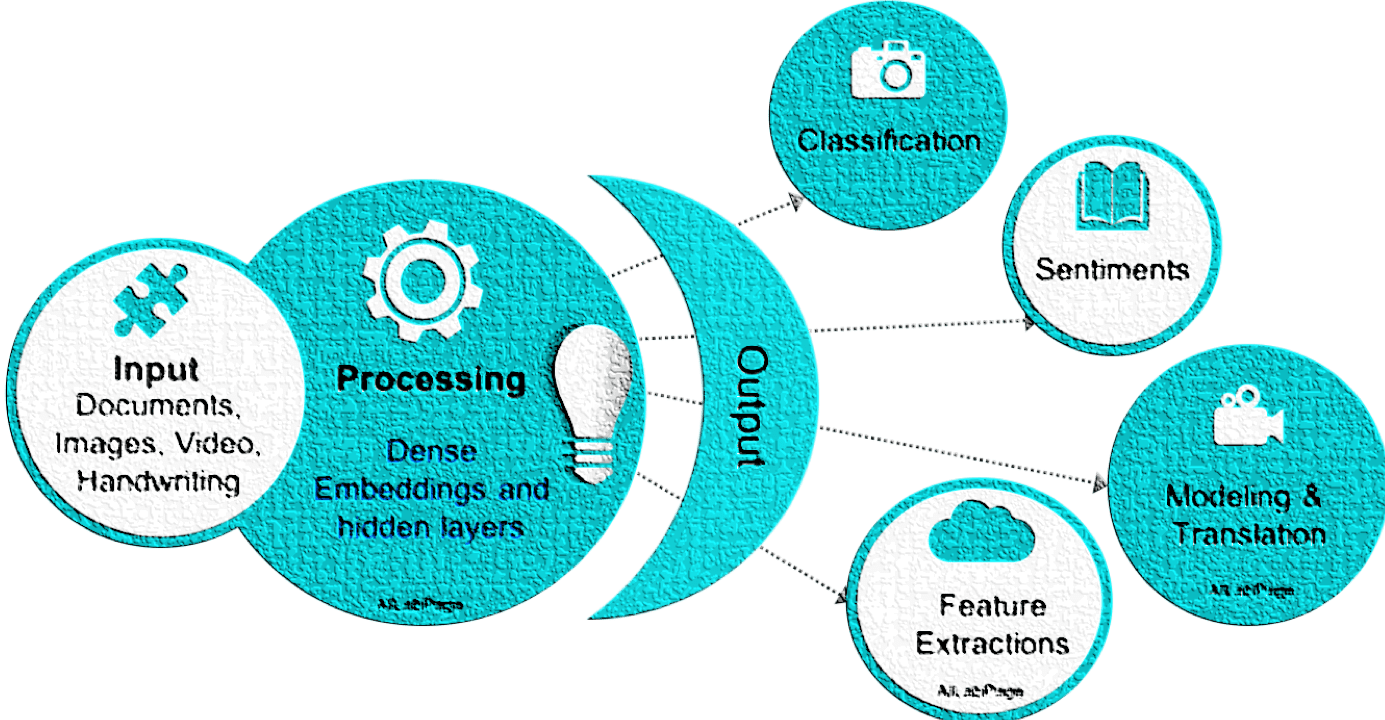### Deep Learning (DL) – Introduction to Basics

AILabPage defines Deep learning is “Undeniably a mind-blowing synchronisation technique applied on data with computing power, skills and experience which practically has no limits“.### Machine Learning – Introduction to Unsupervised Learning

Unsupervised learning is classified as one of the three categories of machine learning, alongside Supervised Machine Learning, and Reinforcement Learning.  Specifically, it falls under the domain of Unsupervised Machine Learning (UML). The predominant technique utilized in the Unified Modeling Language (UML) is cluster analysis. Cluster analysis is employed as a means of discovering concealed patterns or categories within data beyond conventional analytical methods.### How Machine Learning Algorithms Works: An Overview

Machine learning employs computational techniques that empower algorithms to extract valuable insights from data, unconstrained by predefined equations. Through statistical analyses, ML algorithms unravel meaningful patterns and relationships within the data. These discoveries subsequently facilitate automated predictions and decisions, eliminating the need for explicit programming instructions.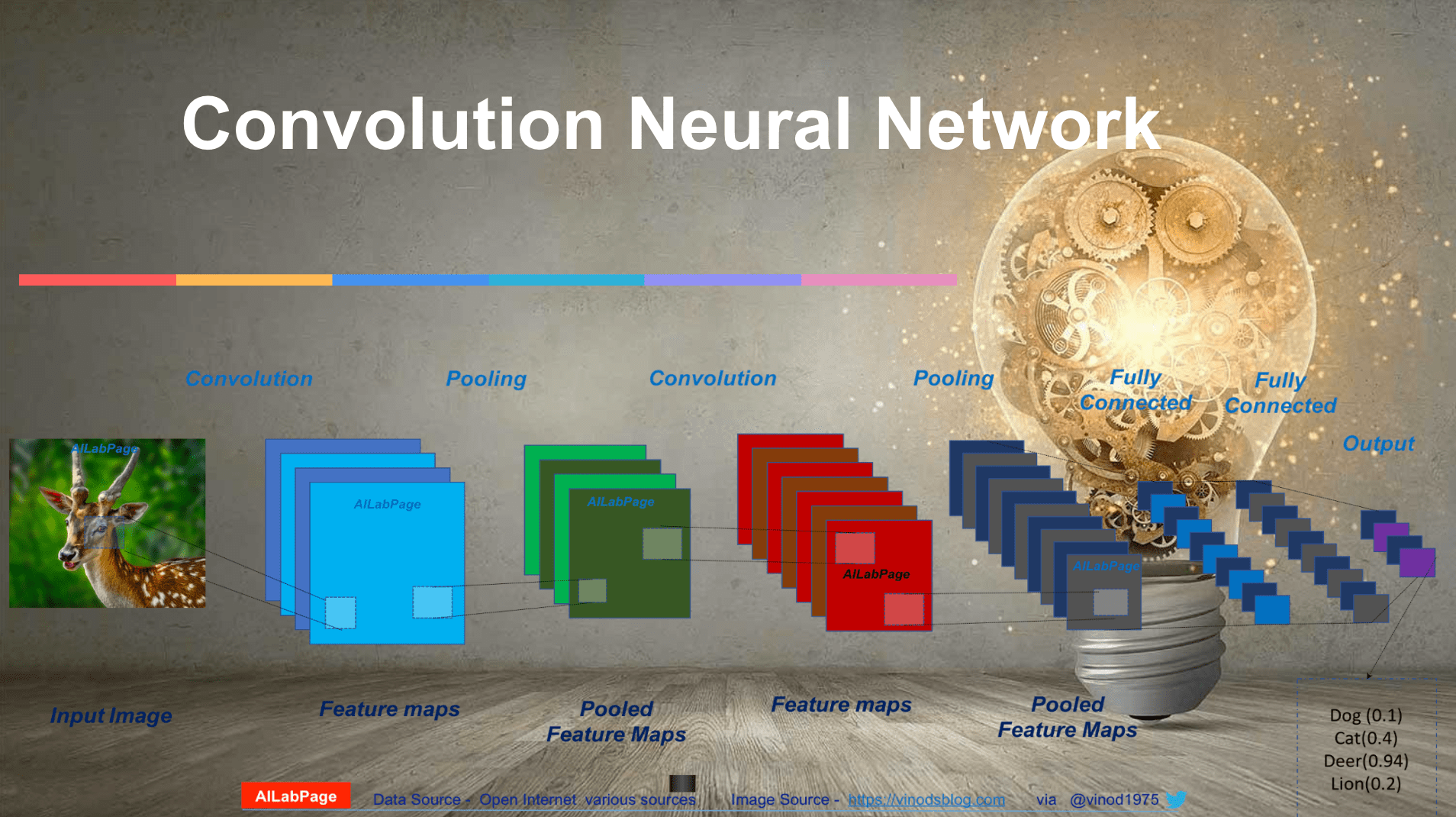### Deep Learning – Introduction to Convolutional Neural Networks

Convolutional neural networks (CNN) are based on simple principles of science and math, even though they may seem complicated to some. When a filter is applied to the input, it will cause the activation to happen. This is a simple and easy thing to do. A feature map is made by using a filter many times on an input. This shows where the features are and how strong they are in the input.### Machine Learning – Introduction to Supervised Learning

AILabPage defines Machine Learning  as “A focal point where business, data and experience meets emerging technology and decides to work together”. SML through historic data set is able to hunt for correct answers, and the task of the algorithm is to find them in the new data. Supervised Machine Learning is